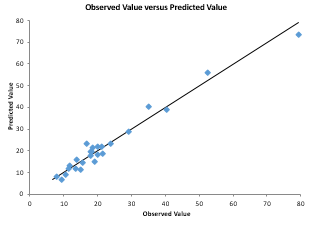•Can I predict the height of children from the height of their mother and father?
• Can I predict product purity from the reaction temperature and reactor residence time?
• Can I predict the hardness of a steel bar from the tempering temperature, bar size and time at temperature?
• Can I predict a student's university grade point average on the basis of their high school grade point average and SAT score?
• Can I predict my ice cream sales based on the temperature and humidity?

Multiple linear regression is used to answer these types of questions by finding if there is a linear relationship between an effect (ice cream sales) and possible causes (temperature and humidity).  The SPC for Excel software contains regression as well as stepwise regression.

## Multiple Linear Regression/Stepwise Regression and SPC for Excel

Multiple linear regression is a method used to model the linear relationship between a dependent variable and one or more independent variables.  It allows you to examine what independent variables (x) impact a response variable (y) and by how much. SPC for Excel contains multiple linear regression that allows you to see if a set of x values impact the response variable.   The output from the SPC for Excel software  includes an in-depth analysis of residuals with potential outliers in red as well as multiple charts to analyze the results. You can easily remove independent variables as well as observations from the data and rerun the regression. You can also transform the y variables.  SPC for Excel also contains stepwise regression.  Stepwise regression is process of building a model by successively adding or removing variables based solely on the p values associated with the t statistic  of their estimated coefficients.   A complete list of regression features is given below.

## Multiple Linear Regression Features

• ANOVA for Model
• Variables Output
• Coefficients
• Standard error
• t statistic, p value
• 95% confidence limit
• VIF
• Standardized coefficients
• Regression statistics
• R2
• Mean, standard error
• Coefficient of variation
• Durbin-Watson statistic
• PRESS
• R2 prediction
• Residuals analysis
• Residuals
• Leverage
• Standardized residuals
• Internally studentized residuals
• Externally studentized residuals
• DFFITS
• Cook's distance
• Standard error of estimated mean
• 95% confidence limits of estimate means
• Potential outliers in red
• Residuals charts (raw, standardized, internally or externally studentized residuals)
• Normal plot
• Versus predicted values
• Versus observation number
• Versus predictor variables
• Other charts
• Predicted vs actual
• DFFITS, Cook's distance and leverage versus observation number
• Remove variables
• Remove observations
• Transform y variables
• p values < 0.05 in red

## Stepwise Regression Features

• Changed p values to enter and to remove variables
• Fit intercept
• Output lists each step of the regression where a variable is added or removed
• Output includes the coefficient, t statistic and p value for each included variable in each step
• Output includes sigma, R2 , and R2 adjusted for each step

#### SPC Knowledge BaseSign up for our FREE monthly publication featuring SPC techniques and other statistical topics.